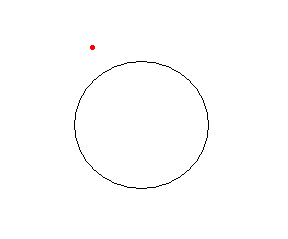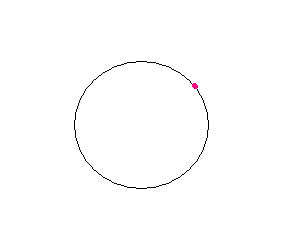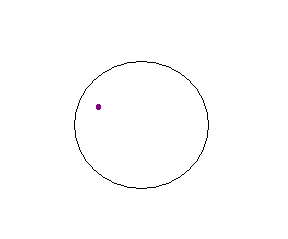# A point in the plane: positions regarding a circumference

When we have a circumference on the plane, a point can be in three different positions regarding the circle we have in the drawing:

• Point outside the circumference

If the distance from the point to the center point of the circumference is greater than the length of the radius.• Point on the circumference

If the distance from the point at the center of the circumference is exactly equal to the length of the radius of the circumference.• Point within the circumference

If the distance from the point to the center of the circumference is less than the length of the radius.